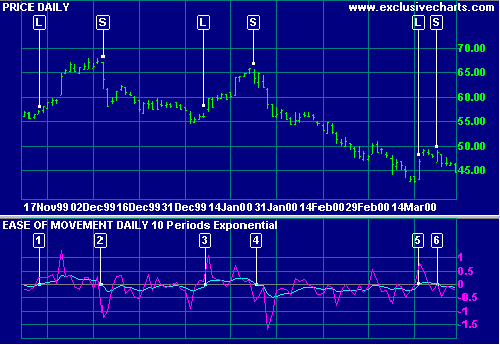# Ease of Movement Indicator

Ease of Movement was developed by Richard W Arms and performs a similar function to Equivolume charts. It highlights the relationship between volume and price changes and is particularly useful for assessing the strength of a trend.

The indicator shows:

• high positive values when prices move upward on light volume;
• high negative values when prices move down on light volume;
• low values if price is not moving or if it takes heavy volume to move prices, signaling distribution or accumulation.

## Ease of Movement Trading Signals

Signals are normally taken from an exponential moving average plotted on the Ease of Movement indicator. They are more effective in a trending market.

• Go long when Ease of Movement crosses to above zero (from below).
• Go short when Ease of Movement crosses to below zero (from above).

### Example

Coca Cola Corporation plotted with   Ease of Movement indicator smoothed by a
10 day exponential moving average.Mouse over chart captions to display trading signals.

1. Go long [L]: Ease of Movement crosses to above zero.
2. Go short [S]: Ease of Movement crosses to below zero.
3. Go long [L]: Ease of Movement crosses to above zero.
4. Go short [S]: Ease of Movement crosses to below zero.
5. Go long [L]: Ease of Movement crosses to above zero.
6. Go short [S]: Note that we are whipsawed more frequently in a ranging market.

### Setup

The Ease of Movement default is set at 10 days smoothing by an exponential moving average. To alter the default settings - Edit Indicator Settings.

See Indicator Panel for directions on how to set up an indicator.

## Ease of Movement Formula

The steps in calculating Ease of Movement are:

1. Calculate the Mid-point for each day:
(High + Low) / 2
2. Calculate the Mid-point Move for each day:
Mid-point [today] - Mid-point [yesterday]
3. The Box Ratio determines the ratio between height and width of the Equivolume box:
Volume [in millions] / (High - Low)
4. Ease of Movement is then calculated as:
Mid-point Move / Box Ratio
5. Ease of Movement is normally smoothed with a 14 day exponential moving average.